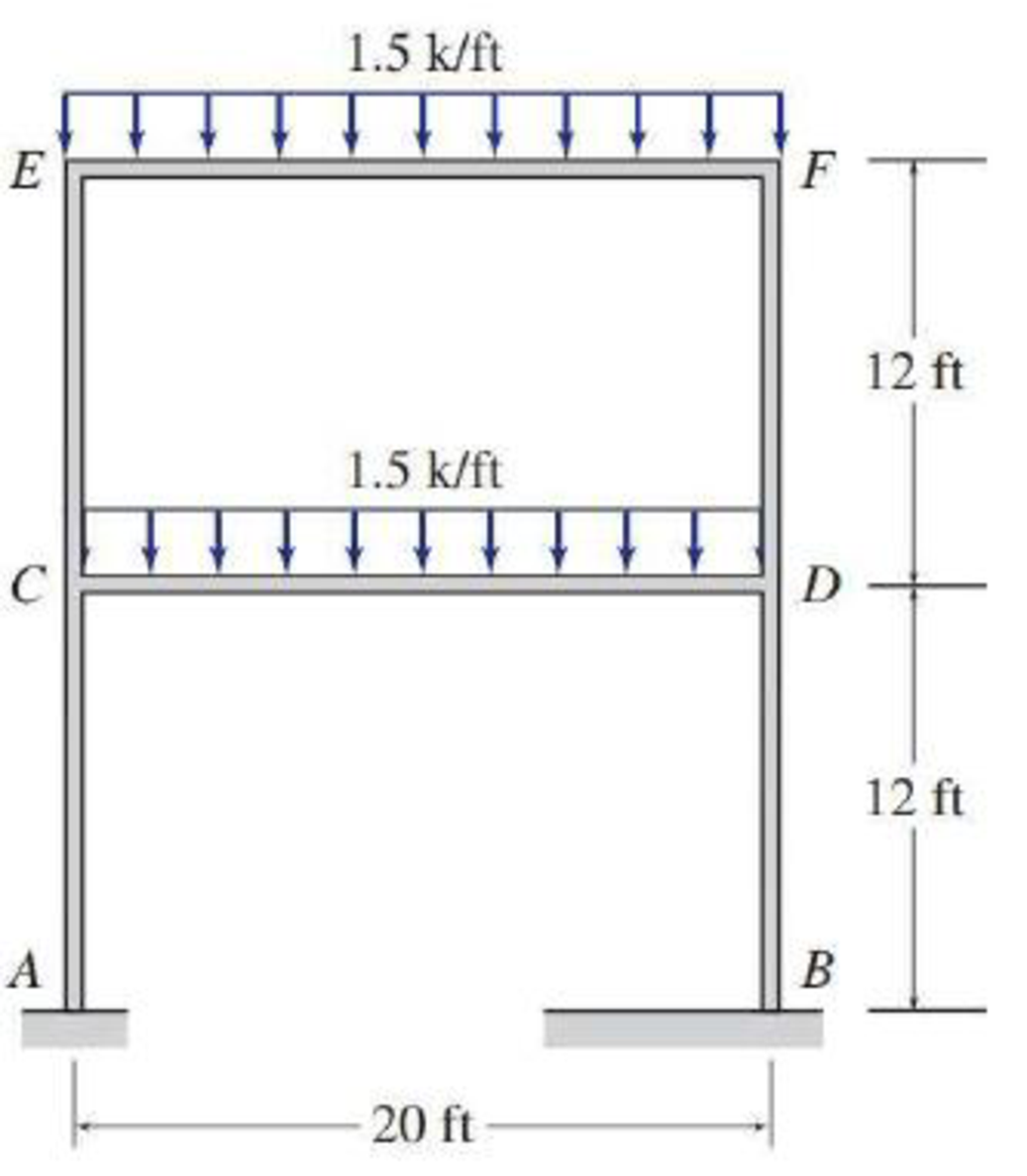# 12.1 through 12.5 Draw the approximate shear and bending moment diagrams for the girders of the frames shown in Figs. P12.1 through P12.5. FIG. P12.2

#### Solutions

Chapter
Section
Chapter 12, Problem 2P
Textbook Problem
197 views

## 12.1 through 12.5 Draw the approximate shear and bending moment diagrams for the girders of the frames shown in Figs. P12.1 through P12.5.FIG. P12.2

To determine

Draw the shear and bending moment diagrams for the girders of given frame.

### Explanation of Solution

Given information:

The uniformly distributed load acting along the girder EF and CD (w) is 1.5 k/ft.

The horizontal distance of the member EF and CD (L) is 20 ft.

The vertical distance of the members AC, CE, BD, and DF (lv) is 12 ft.

Calculation:

The span length and loads for the two girders of the frame EF and CD are same; therefore the approximate shear and bending moment diagrams for the girders will also be the same.

Determine the span for the middle portion of the girder using the relation.

lm=0.8L

Substitute 20 ft for L.

lm=0.8×20=16ft

Determine the span for the two end portion of the girder using the relation.

le=0.1L

Substitute 20 ft for L.

le=0.1×20=2ft

Draw the statically determinate girder portion as in Figure (1).

Consider the equilibrium of the simply supported middle portion of the girder.

Determine the vertical reactions at the end portion using the relation.

V=wlm2

Substitute 1.5 k/ft for w and 16 ft for lm.

V=1.5×162=12k

Consider the equilibrium conditions of the end portions of the girder.

Consider upward direction is positive and counter clockwise moment is positive.

Determine the support reaction at the left end.

Apply the equations of equilibrium to the left end portion.

FY=0SLw×leV=0

Substitute 1.5 k/ft for w, 2 ft for le, and 12 k for V.

SL1.5×212=0SL=15k()

Determine the moment at the left end.

Take moment about left end is equal to zero.

ML=0MLw×le×le2V×le=0

Substitute 1

### Still sussing out bartleby?

Check out a sample textbook solution.

See a sample solution

#### The Solution to Your Study Problems

Bartleby provides explanations to thousands of textbook problems written by our experts, many with advanced degrees!

Get Started

Find more solutions based on key concepts
Investigate the characteristics of titanium alloys used in medical implants for hips and other joint replacemen...

Engineering Fundamentals: An Introduction to Engineering (MindTap Course List)

What are the two main components of the motherboard? (110)

Enhanced Discovering Computers 2017 (Shelly Cashman Series) (MindTap Course List)

What is data analytics? Briefly define explanatory and predictive analytics.

Database Systems: Design, Implementation, & Management

What is calibration and why is it important?

Precision Machining Technology (MindTap Course List)

Convert 10,000 ft-lbf of energy into BTU, Joules, and kilojoules.

Fundamentals of Chemical Engineering Thermodynamics (MindTap Course List)

If your motherboard supports ECC DDR3 memory, can you substitute non-ECC DDR3 memory?

A+ Guide to Hardware (Standalone Book) (MindTap Course List)